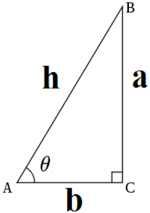# 三角函数

## 三角公式{\displaystyle {\theta}}正弦是对边与斜边的比值：{\displaystyle \sin {\theta }={\frac {a}{h}}}

{\displaystyle \theta }余弦是邻边与斜边的比值：{\displaystyle \cos {\theta }={\frac {b}{h}}}

{\displaystyle \theta }正切是对边与邻边的比值：{\displaystyle \tan {\theta }={\frac {a}{b}}}

{\displaystyle \theta }余切是邻边与对边的比值：{\displaystyle \cot {\theta }={\frac {b}{a}}}

{\displaystyle \theta }正割是斜边与邻边的比值：{\displaystyle \sec {\theta }={\frac {h}{b}}}

{\displaystyle \theta }余割是斜边与对边的比值：{\displaystyle \csc {\theta }={\frac {h}{a}}}

• 航海学
• 测绘学
• 工程学